# Lorene::Change_var Class Reference [General PDE solvers (under development)]

This class defines a variable change to be used when solving elliptic equations. More...

#include <change_var.h>

List of all members.

## Public Member Functions

Change_var (int var)
Additive factor for F ## PROVISORY.
Change_var (int var, double)
Standard constructor.
Change_var (int var, double, double)
Standard constructor.
Change_var (const Change_var &so)
~Change_var ()
Constructor by copy.
double val_F (double x)
Standard destructor.
double val_der_F (double x)
Returns the value of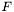at { x}.
double val_G (double x)
Returns the value of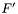at { x}.
double val_der_G (double x)
Returns the value of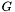at { x}.

## Protected Attributes

double(* func_F )(double)
double(* der_F )(double)
Pointer on the function.
double(* func_G )(double)
Pointer on the derivative of.
double(* der_G )(double)
Pointer on the function.
double mult_F
Pointer on the derivative of.
Multiplicative factor for F ## PROVISORY.

## Detailed Description

This class defines a variable change to be used when solving elliptic equations.

In {one particular domain}, the change is defined as follows : ()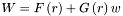where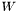is the usual variable, i.e. the one that is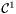and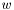is the variable used for solving the elliptic equation. The functionsandare arbitrary functions of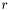. The type of change must be explicitely implemented in the constructor for { Change_var}.

Definition at line 98 of file change_var.h.

## Constructor & Destructor Documentation

 Lorene::Change_var::Change_var ( int var )

Additive factor for F ## PROVISORY.

Standard constructor. Case with without parameter. { var} defines explicitely the type of variable to be used. Are currently implemented : {itemize} { var}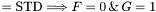. { var}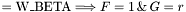. { var}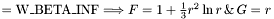. { var}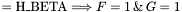. { var}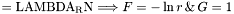. { var}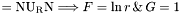. {itemize}

Definition at line 102 of file change_var.C.

References add_F, der_F, der_G, func_G, and mult_F.

 Lorene::Change_var::Change_var ( int var, double mult )

Standard constructor.

Case with one multiplicative parameter for F { var} defines explicitely the type of variable to be used.

Definition at line 160 of file change_var.C.

References add_F, der_F, der_G, func_G, and mult_F.

 Lorene::Change_var::Change_var ( int var, double mult, double add )

Standard constructor.

Case with one multiplicative parameter for F and one additive. { var} defines explicitely the type of variable to be used.

Definition at line 217 of file change_var.C.

References add_F, der_F, der_G, func_G, and mult_F.

 Lorene::Change_var::~Change_var ( )

Constructor by copy.

Definition at line 277 of file change_var.C.

## Member Function Documentation

 double Lorene::Change_var::val_der_F ( double x )

Returns the value ofat { x}.

Definition at line 283 of file change_var.C.

References der_F, and mult_F.

 double Lorene::Change_var::val_der_G ( double x )

Returns the value ofat { x}.

Definition at line 291 of file change_var.C.

References der_G.

 double Lorene::Change_var::val_F ( double x )

Standard destructor.

Definition at line 279 of file change_var.C.

 double Lorene::Change_var::val_G ( double x )

Returns the value ofat { x}.

Definition at line 287 of file change_var.C.

References func_G.

## Member Data Documentation

 double Lorene::Change_var::add_F [protected]

Multiplicative factor for F ## PROVISORY.

Definition at line 107 of file change_var.h.

 double(* Lorene::Change_var::der_F)(double) [protected]

Pointer on the function.

 double(* Lorene::Change_var::der_G)(double) [protected]

Pointer on the function.

 double(* Lorene::Change_var::func_G)(double) [protected]

Pointer on the derivative of.

 double Lorene::Change_var::mult_F [protected]

Pointer on the derivative of.

Definition at line 106 of file change_var.h.

The documentation for this class was generated from the following files:

Generated on 7 Dec 2019 for LORENE by1.6.1